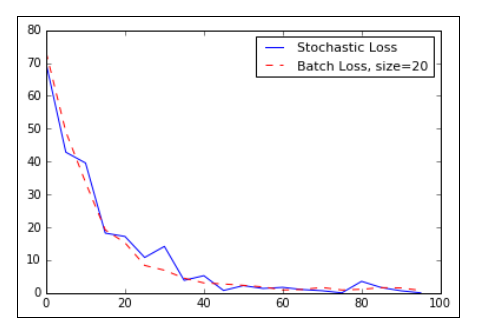# TensorFlow with Batch and Stochastic Training

Original article was published by Bhanu Soni on Deep Learning on Medium# TensorFlow with Batch and Stochastic Training

TensorFlow updates our model variables according to the prior described backpropagation, it can operate on anywhere from one datum observation to a large group of data at once. Operating on one training example can make for a very erratic learning process while using a too large batch can be computationally expensive. Choosing the right type of training is crucial to getting our machine learning algorithms to converge to a solution.

1. In order for TensorFlow to compute the variable gradients for backpropagation to work, we have to measure the loss on a sample or multiple samples.
2. Stochastic training is only putting through one randomly sampled data-target pair at a time.
3. Another option is to put a larger portion of the training examples in at a time and average the loss for the gradient calculation.
4. Batch training size can vary up to and including the whole dataset at once.

We will show how to extend the prior regression example(TensorFlow Backpropagation), which used stochastic training to batch training.

Let’s begin with by loading NumPy , matplotlib , and TensorFlow and start a graph session, as
follows:

`import matplotlib as pltimport numpy as npimport tensorflow as tfsess = tf.Session()`

# How to do it…

1. We will start by declaring a batch size. This will be how many data observations we will feed through the computational graph at one time:
`batch_size = 20`

2. Next we declare the data, placeholders, and the variable in the model. The change we make is the shape of the placeholders. They are now two dimensions, where the first dimension is None, and second will be the number of data points in the batch. We could have explicitly set it to 20, but we can generalize and use the None value.

`x_vals = np.random.normal(1, 0.1, 100)y_vals = np.repeat(10., 100)x_data = tf.placeholder(shape=[None, 1], dtype=tf.float32)y_target = tf.placeholder(shape=[None, 1], dtype=tf.float32)A = tf.Variable(tf.random_normal(shape=[1,1]))`

3. Now we add our operation to the graph, which will now be matrix multiplication instead of regular multiplication. Remember that matrix multiplication is not communicative so we have to enter the matrices in the correct order in the matmul() function:

`my_output = tf.matmul(x_data, A)`

4. Our loss function will change because we have to take the mean of all the L2 losses of each data point in the batch. We do this by wrapping our prior loss output in TensorFlow’s reduce_mean() function:

`loss = tf.reduce_mean(tf.square(my_output - y_target))`

5.We declare our optimizer just like we did before:

`my_opt = tf.train.GradientDescentOptimizer(0.02)train_step = my_opt.minimize(loss)`

6. Finally, we will loop through and iterate on the training step to optimize the algorithm. This part is different than before because we want to be able to plot the loss over versus stochastic training convergence. So we initialize a list to store the loss function every five intervals:

`loss_batch = []for i in range(100):    rand_index = np.random.choice(100, size=batch_size)    rand_x = np.transpose([x_vals[rand_index]])    rand_y = np.transpose([y_vals[rand_index]])    sess.run(train_step, feed_dict={x_data: rand_x, y_target:rand_y})    if (i+1)%5==0:        print('Step #' + str(i+1) + ' A = ' + str(sess.run(A)))        temp_loss = sess.run(loss, feed_dict={x_data: rand_x, y_target: rand_y})        print('Loss = ' + str(temp_loss))        loss_batch.append(temp_loss)`

7. Here is the final output of the 100 iterations. Notice that the value of A has an extra dimension because it now has to be a 2D matrix:

`Step #100 A = [[ 9.86720943]]Loss = 0.`

# How it works…

1. Batch training and stochastic training differ in their optimization method and their convergence.
2. Finding a good batch size can be difficult.
3. To see how convergence differs between batch and stochastic, here is the code to plot the batch loss from above.
4. here is also a variable here that contains the stochastic loss, but that computation follows from the previous article.
5. The code to save and record the stochastic loss in the training loop. Just substitute this code in the prior code
`loss_stochastic = []for i in range(100):    rand_index = np.random.choice(100)    rand_x = [x_vals[rand_index]]    rand_y = [y_vals[rand_index]]    sess.run(train_step, feed_dict={x_data: rand_x, y_target: rand_y})    if (i+1)%5==0:        print('Step #' + str(i+1) + ' A = ' + str(sess.run(A)))        temp_loss = sess.run(loss, feed_dict={x_data: rand_x, y_target: rand_y})        print('Loss = ' + str(temp_loss))        loss_stochastic.append(temp_loss)`

The plot of both the stochastic and batch loss for the same regression problem:

`plt.plot(range(0, 100, 5), loss_stochastic, 'b-', label='StochasticLoss')plt.plot(range(0, 100, 5), loss_batch, 'r--', label='Batch' Loss,size=20')plt.legend(loc='upper right', prop={'size': 11})plt.show()`